# Maharashtra Board Class 9 Science Solutions Chapter 12 Study of Sound

Balbharti Maharashtra State Board Class 9 Science Solutions Chapter 12 Study of Sound Notes, Textbook Exercise Important Questions and Answers.

## Maharashtra State Board Class 9 Science Solutions Chapter 12 Study of Sound

Class 9 Science Chapter 12 Study of Sound Textbook Questions and Answers

1. Fill in the blanks and explain.

a. Sound does not travel through ……………………….……….. .
b The velocity of sound in steel is ……………………….………… than the velocity of sand in water.
c. The incidence of ……………………….………… in daily life shows that the velocity of sound is less than the velocity of light.
d. To discover a sunken ship or objects deep inside the sea, ……………………….………… technology is used.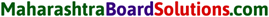2. Explain giving scientific reasons.

a. The roof of a movie theatre and a conference hall is curved.

• Sound waves get reflected from the walls and roof of a room multiple times. This causes a single sound to be heard not once but continuously. This is called reverberation.
• Due to reverberation, some auditoriums or some particular seats in an auditorium have inferior sound reception. This can be compensated with curtains.
• Ceilings of these halls are made curved so that sound after reflecting from the ceiling, reaches all parts of the hall and the quality of sound improves.

b. The intensity of reverberation is higher in a closed and empty house.

• Reverberation occurs due to multiple reflections of sound.
• The furniture in the house acts as a sound-absorbing material.
• So if the house is closed and empty, a reflection of sound will be maximum and hence, intensity of reverberation is higher.

c. We cannot hear the echo produced in a classroom.

• For distinct echoes, the minimum distance of the reflecting surface from the source of sound must be 17.2 m.
• Benches in the classroom are sound absorbing materials which prevent echo of sound.
• Because of these two reasons echo is not heard in a classroom.

a. What is an echo? What factors are important to get a distinct echo?

• An echo is the repetition of the original sound because of reflection by some surface.
• At 22°C, the velocity of sound in air is 344 m/s.
• Our brain retains a sound for 0.1 seconds Thus, for us to be able to hear a distinct echo, the sound should take more than 0.1 seconds after starting from the source to get reflected and. come back to us.
• We know that,
Distance = speed x time
= 344 m/s x 0.1 s
= 34.4 m
• Thus, to be able to hear a distinct echo, the reflecting surface should be at a minimum distance of half of the above, i.e. 17.2 m.
• As the velocity of sound depends on the temperature of air, this distance depends on the temperature.b. Study the construction of the Golghumat at Vijapur and discuss the reasons for the multiple echoes produced there.

• Goighumat with a height of 51 metres and diameter of 37 metres with 3 metres thick walls is spread over approximately 1700 square metres.
• This meets the conditions for echo i.e. : 17.2 metres minimum.
• The dome of the golghumat is curved and hence, sound reflects multiple times before reaching the observer.
• This is the reason for multiple echoes being produced.

c. What should be the dimensions and the shape of classrooms so that no echo can be produced there?

1. Dimensions: The distance between opposite walls in a classroom must be less than 17.2 m so that the reflected sound returns to the observer within 0.1 s.
2. Shape: The classrooms should have curved ceilings and walls so that the reflected sound is directed towards the observer instantly within 0.1 s

4. Where and why are sound-absorbing materials used?
The sound absorbing materials are used in :

• School, cinema hall, concert hall, houses or places where quality of sound is important.
• In the absence of sound absorbing material the sound will undergo multiple reflection causing reverberation of sound.

5. Solve the following examples.

a. The speed of sound in air at O °C is 332 m/s. If it increases at the rate of 0.6 m/s per degree, what will be the temperature when the velocity has increased to 344 m/s?
Given:
Initial speed of sound at 0°C 332 m/s.
Final speed of sound -344 m/s.
Rate of increase per degree rise in temp. = 0.6m/s
To find:
Temperature when speed is 344m/s
Formulae:
Increase in temperature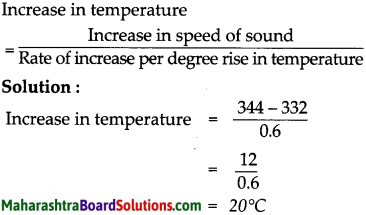Temperature when the speed of sound is 344 m/sis 20°Cb. Nita heard the sound of lightning after 4 seconds of seeing it. What was the distance of the lightning from her? (The velocity of sound in air is 340 m/s?)
Given : Speed of sound (v) = 340 m/s
Time taken (f) = 4 sec
To find : Distance (s) = ?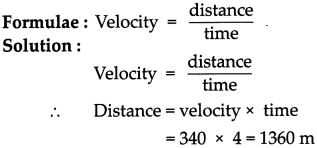The lightning has struck at a distance of 1360 m from the observer.

c. Sunil is standing between two walls. The wall closest to him is at a distance of 360 m. If he shouts, he hears the first echo after 4 s and another after another 2 seconds.
1. What is the velocity of sound in air?
2. What is the distance between the two walls? (Ans: 330 m/s; 1650 m)
Given:
Distance of the closer wall (S1) = 660 m
Time of echo from closer wall = 4 sec
∴ Time taken (t1) = 4/2 sec = 2 sec
Time of echo from distant wall = 6 sec
∴ Time taken (t2) = 6/2 sec = 3 sec
To find :
Velocity of sound in air (y) =?
Distance between two walls (S1 + S2) = ?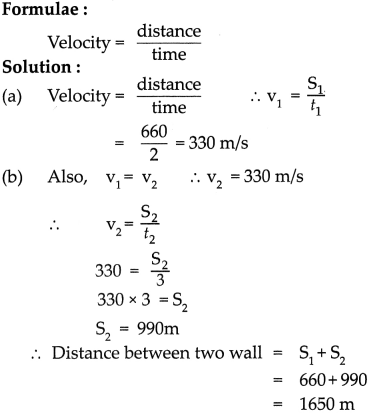The velocity of sound in air is 330 mIs and the distance between two walls is 1650 m.d. Hydrogen gas is filled in two identical bottles, A and B, at the same temperature. The mass of hydrogen in the two bottles is 12 gm and 48 gm respectively. In which bottle will sound travel faster? How may times as fast as the other? (Ans: In A; Twice)
In A; Thrice

e. Helium gas is filled in two identical bottles A and B. The mass of the gas in the two bottles is 10 gm and 40 gm respectively. If the speed of sound is the same in both bottles, what conclusions will you draw? (Ans: Temperature of B is 4 times the temperature of A.)
Given:
Mass of Helium in bottle A = (mA) = 10gm
Mass of Helium in bottle B = (mB) = 40gm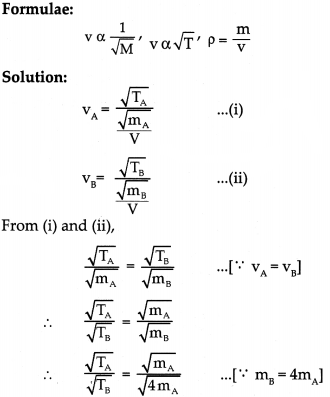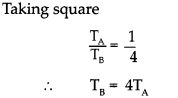The temperature of B is 4 times the temperature of A

Class 9 Science Chapter 12 Study of Sound Intext Questions and Answers

Study Of Sound Class 9 Notes Maharashtra Board Question 1.
How does the velocity of sound depend on its frequency?
The velocity of sound is directly proportional to its frequency
ν = υ λ
when ν = velocity
υ = frequency
λ = wavelength

9th Class Science Chapter 12 Study Of Sound Exercise Question 2.
The molecular weight of oxygen gas (O2) is 32 while that of hydrogen gas (H2) is 2. Prove that under the same physical conditions, the velocity of sound in hydrogen is four times that in oxygen.
Given:
Molecular wt of Oxygen (Mo) =32
Molecular wt of hydrogen (MH) = 2
To Find:
VH = 4 vo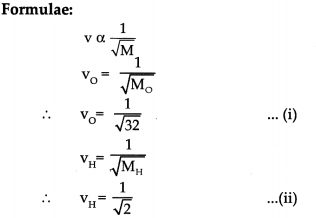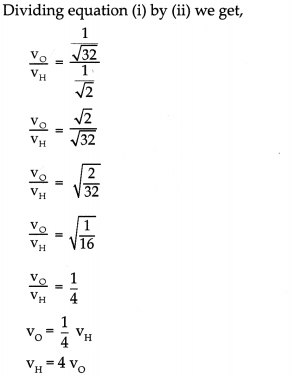Hence, proved that velocity of sound in hydrogen is four times that in oxygen.Study Of Sound Class 9 Maharashtra Board  Question 1.
How will you reduce reverberation in public halls or buildings?
(i) Reverberation in public halls or buildings will be reduced by using sound absorbing materials like curtains on wall, carpets on the floor.
(ii) By keeping the windows open, as sound will not get reflected.

12 Study Of Sound 9th Class Exercise  Question 2.
How is ultrasound used in medical science?

• Sonography: Sonography technology uses ultrasonic sound waves to generate images of internal organs of the human body.
• Echocardiography: Echocardiography is a test that uses ultrasonic sound waves to produce live images of your heart.

9th Science Chapter 12 Study Of Sound Exercise Question 3.
To hear the echo distinctly, will the distance from the source of sound to the reflecting surface be same at all temperatures? Explain your answer.

• No,the distance from the source of sound to the reflecting surface will not be the same at all temperatures.
• Velocity of sound is directly proportional to the square root of temperature.
• One of the conditions of echo is that the time interval between the original and reflected sound should be more than 0.1 sec.
• So if the temperature increases, the velocity of sound increases and the reflected sound reaches in less than 0.1 sec.
• So for echo to be heard the distance between the observer and the reflecting surface has to increase.

9th Science Chapter 12 Study Of Sound Question 4.
When is the reflection of sound harmful?

• Reflected sound of high intensity called as noise is disturbing and harmful to the ears.
• When sound reverberates i.e it undergoes multiple reflections, poor quality of sound is produced.

9th Class Science Chapter 12 Study Of Sound Question Answer Question 5.
What kind of waves are created when a stone is dropped in water ?

• When a stone is dropped in water, the particles of water oscillate up and down.
• These oscillations are perpendicular to the direction of propagation of the wave, such waves are called transverse waves.12 Study Of Sound 9th Class Question 1.
Observe the graph/ diagram and discuss your observation.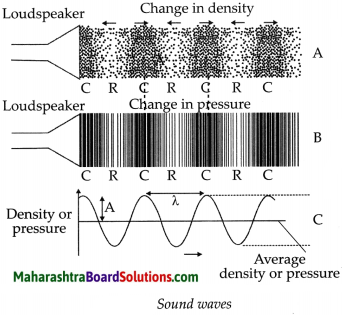1. Fig. A shows changes in density. The region where particles are crowded is called compression and where they are far apart are rarefaction.
2. Fig. B show change in pressure. The lines represent layers of air. The regions when lines are crowded are high pressure regions while when they are far apart are of low pressure.
3. Fig. C shows changes in density or pressure. The crest represents high pressure region while trough represents low pressure region.

Study Of Sound Class 9 Question Answer Question 1.
How are the frequencies of notes sa, re, ga, ma, pa, dha, ni related to each other?
The frequencies of notes sa, re, ga, ma, pa, dha, ni are related in the ratio.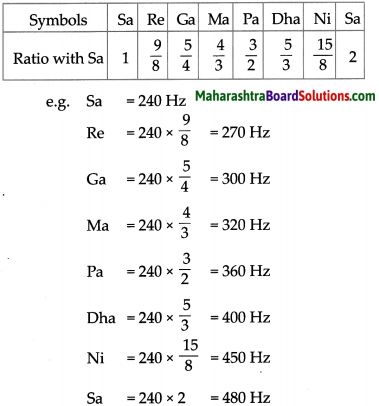i.e if first Sa is 240Hz then the next Sa will be 240 x 2 = 480HzClass 9 Science Chapter 12 Study Of Sound Exercise Question 2.
What is the main difference between the frequencies of the voice of a man and that of a woman?

• Voice of a woman is high pitch i.e shorter wavelength and higher frequency
• Voice of man is low pitch i.e larger wavelength and smaller frequency.

Question 3.
Try this;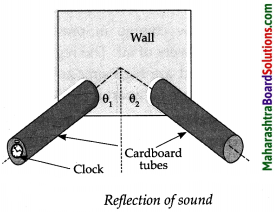(a) In the above activity, what will happen if you lift one of the tubes to some height?
If one of the tubes is lifted, angle of incidence will not be equal to angle of reflection, hence, the sound will not be clearly audible.

(b) Measure the angle of incidence 01 and the angle of reflection 02. Try to see if they are related in any way.
Angle of incidence is same as the angle of reflection.

Class 9 Science Chapter 12 Study of Sound Additional Important Questions and Answers

Can you recall?

12.Study Of Sound Question 1.
How is the direction of the oscillation of the particles of the medium related to the direction of propagation if the sound wave?

• Sound travels as a longitudinal wave.
• In a longitudinal wave, the particle of the medium oscillate parallel to the direction of propagation of the wave.

Choose and write the correct option:

Class 9 Science Chapter 12 Study Of Sound Question 1.
The unit of frequency is ……………………………… .
(a) Hertz
(b) m/s2
(c) Decibels
(d) m/s
(a) Hertz

Study Of Sound Class 9 Exercise Question 2.
The normal hearing range for humans is ……………………………… .
(a) 0 Hz to 20 Hz
(b) greater than 20,000 Hz
(c) 20 Hz to 20,000 Hz
(d) none of these
(c) 20 Hz to 20,000 HzClass 9th Science Chapter 12 Study Of Sound Question Answer  Question 3.
Sound will not travel through ……………………………… .
(a) Vacuum
(b) Liquid
(c) Solid
(d) Gases
(a) vacuum

Class 9 Science Chapter 12 Study Of Sound Question Answer Question 4.
SI unit of ………………………………. is Hertz (Hz).
(a) Wavelength
(b) Frequency
(c) Speed of wave
(d) Velocity
(b) frequency

Reflection Of Sound Class 9 Question 5.
The velocity of sound is inversely proportional to the ……………………………… .
(a) Pressure
(b) Square root of temperature
(c) Square root of density
(d) Humidity
(c) square root of density

Question 6.
Sound waves with frequency greater than 20 kHz are called ……………………………… .
(a) Infrasound
(b) Ultrasound
(c) Sonic
(d) Damped sound
(b) ultrasoundQuestion 7.
The loudness of a sound depends upon ……………………………… .
(a) Amplitude
(b) Speed
(c) Density
(d) Wavelength
(a) Amplitude

Question 8.
……………………………… are used in sonography.
(a) High frequency ultrasound
(b) Stationary waves
(c) High frequency infrasound
(d) High frequency micro waves
(a) High frequency ultrasound

Question 9.
The ……………………………… receives the vibrations coming from the membrane and converts them into electrical signals which are sent to the brain through the nerve.
(a) Cochlea
(b) Tympanic cavity
(c) Stapes
(d) Pinna
(a) Cochlea

Find the odd one out:

Question 1.
Bats, rats, cats, dolphins
Cats: cannot produce ultrasonic sound.Question 2.
Clothes, paper, curtains, mirror
Mirror: is a good reflector of sound, while others are poor reflectors.

Question 3.
Submarines, icebergs, internal organ, sunken ships.
Internal organ: sonography is used , while for others sonar system is used.

Question 4.
Temperature, density, molecular weight, pressure
Pressure: for a fixed temperature, the speed of sound does not depend on the pressure of the gas, all other factors affect speed of sound.

Question 1.
How can one produce sound?
Vibration set up in an object produces sound (or) sound is produced when an object is disturbed and starts vibrating.

Question 2.
What is velocity of sound wave ?
The distance covered by a point on the wave in unit time is the velocity of the sound wave.

Question 3.
What is the minimum distance of the reflecting surface to hear an echo ?
To be able to hear a distinct echo, the reflecting surface should be at a minimum distance of 17.2 m.Match the columns:

Question 1.

 Column ‘A’ Column B’ Column C (1) Transverse wave (a) Particles oscillate parallel to direction of propagation (i) Wave produced in a slinky (2) Longitudinal wave (b) Particles oscillate perpendicular to direction of propagation (ii) Frequency less than 20 Hz (3) Ultrasound (c) Echo formation is heard under particular conditions (iii) Wave produced in string (4) Infrasound (d) High frequency waves (iv) Frequency between 20 Hz to 20000 Hz (5) Audible frequency (e) Low frequency waves (v) Frequency greater than 20000 Hz

(1-b- iii),
(2a- i),
(3 – d – v),
(4 – e – ii),
(5 -c- iv)

Question 2.

 Column A’ Column ‘B’ Column C (1) Amplitude (a) T (i) Pitch of sound (2) Frequency (b) A (ii) Loudness of sound (3) Wavelength (c) υ (iii) Reciprocal of frequency (4) Time period (d) λ (iv) v/υ

(1 -b – ii),
(2 -c – i),
(3-d – iv),
(4 – a – iii)Name the following:

Question 1.
A form of energy which produces sensation of hearing in our ears.
Sound energy

Question 2.
Repetitions of sound due to reflection .
Echo

Question 3.
The audible range of sound for human being.
20 Hz to 20,000 Hz

Question 4.
A method to obtain images of internal organs of the human body.
Sonography

Question 5.
The matter or substance through which sound gets transmitted.
Solid, liquid, gases

Question 6.
Three major parts of the ear.
External ear, the middle ear and the inner ear.

Question 7.
Any two examples in which infrasound is produced.
Pendulum, earthquake.Question 8.
Name the living beings that can produce ultrasound.
Bats, dolphins, mice.

Give scientific reasons:

Question 1.
Bats can navigate in dark.

• The ultrasonic sound produced by bats, gets reflected on hitting an obstacle.
• This reflected sound is received by their ears and they can locate the obstacle and estimate its distance even in the dark.
• Hence, bats can navigate in dark.

Question 2.
A SONAR system is installed in a ship.

• A SONAR system determines the depth of the sea.
• It locates underwater hills, valleys, icebergs, submarines and sunken ships. It also locates the positions of other ships or submarines.
• Hence a SONAR system is installed in a ship.

Question 3.
Sound travels faster in iron than in air.

• Sound requires a material medium for its propagation and travels in the form of a longitudinal wave.
• The denser the medium, faster is the propagation of sound.
• Hence, sound travels faster in iron than in air.Solve the following:

Type – A

Formula:
$$\text { (i) Velocity }=\frac{\text { distance }}{\text { time }}$$

Question 1.
Ultrasonic waves are transmitted downwards into the sea with the help of a SONAR. The reflected sound is received after 4 s. What is the depth of the sea at that place? (Velocity of sound in seawater = 1550 m/s)
Given:
Time to hear echo = 4 sec
Time taken by sound waves to reach the bottom 4 of sea (t) = 4/2 sec = 2 sec
Velocity of sound in sea water (v) = 1550 m/s
To find:
Depth of sea(s) = ?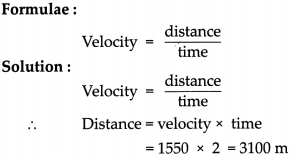The depth of the sea at that place is 3100 m.

Question 2.
A person standing near a hill fires a gun and hears the echo after 1 second. If speed of sound in air is 340 m/s. Find the distance between the hill and the person.
Given:
Time to hear echo = 1 sec 1
Time taken (t) = 1/2 sec
Velocity of sound (v) = 340 m/s
To find:
Distance (s) = ?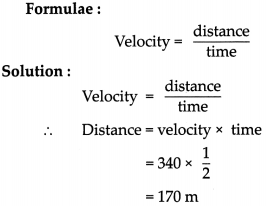Distance between the person and hill is 170 m.Numerical For Practice

Question 3.
If you hear the thunder 20 seconds after you see the flash of lightning, how far from you has the lightning occurred? (Speed of sound in air = 340 m/s)
6800m

Question 4.
Aboy observes smoke from a cannon 3 seconds before he hears the bang. If the cannon is 1020 m from the observer, find the velocity of sound.
340 rn/s

Question 5.
A soldier standing between the two buildings fires a gun. He heard the echo of the sounds from the first building after 2 seconds and echo from the second building after 3 seconds. Find the distance between two buildings. (Speed of sound in air = 340 m/s)
850m

Type – B

$$Formula: (i) Velocity = Frequency \times Wavelength (ii) Velocity =\frac{\text { distance }}{\text { time }}$$

Question 1.
Sound waves of wavelength 1 cm have a velocity of 340 mIs in air. What is their frequency? Can this sound be heard by the human ear?
Given:
wave length (λ) = 1cm = 1/100
Velocity of sound (v) = 340 m/s
To fInd :
frequency (u) = ?
Formulae:
ν = υ λ
Solution: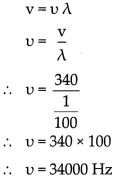The frequency of the sound waves is 34000 Hz. The frequency is higher than 20000 Hz and therefore, this sound cannot be heard by the human ear.Question 2.
How long will it take for a sound wave of 25 cm wavelength and 1.5 kHz frequency, to travel a distance of 1.5 km?
Given:
frequency (u) = 1.5 kHz = 1500 Hz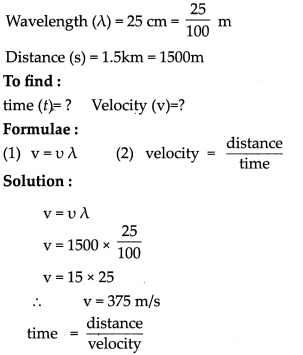$$\begin{array}{l} =\frac{1500}{375} \\ =4 \mathrm{sec} \end{array}$$
The sound wave takes 4 sec to travel the distance of 1.5 km.

Question 3.
Calculate distance travelled by a sound wave having frequency 1000 Hz and wavelength 0.25 m, if it travels for 5 seconds in a certain medium.
Given:
frequency (u) = 1000 Hz
wavelength (λ) = 0.25 m
time (t) = 5 seconds
To find :
Distance (d) =?
Formulae:
ν = υ λ
Solution: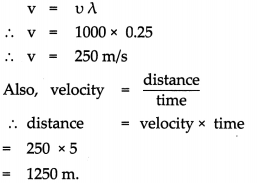The distance travelled by the sound wave is 1250 m.Question 4.
The audible range of sound is 20 Hz to 20000 Hz. At 22°C in air speed of sound is 344 mIs. Express the range of sound in terms of wavelength by calculating the respective values.
Given:
frequency (u1) 20 Hz
frequency ( u2) = 20,000 Hz
velocity (v) = 344 rn/s
To find :
Wavelengths λ1 and λ2 = ?
Formulae:
ν = υ λ
Solution: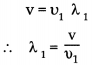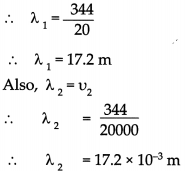Audible range of wavelength of sound is from 17.2 x 10-3 m to 17.2 m.

Numerical For Practice

Question 5.
A sound wave has frequency 320 Hz and wavelength 0.25 m. How much distance will it travel in 10 second?
The distance travelled is 800 m.

Type – C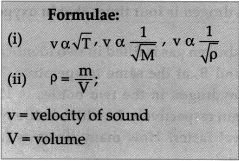Question 1.
Hydrogen gas is filled in two identical bottles, A and B, at the same temperature. The mass of hydrogen in the two bottles is 12 gm and 48 gin respectively. In which bottle will sound travel faster? How many timés as fast as the other?
Given:
Mass of hydrogen in bottle A (mA) = 12gm
Mass of hydrogen in bottle B(mB) = 48gm
To find:
In which bottle sound travels faster.
Formulae: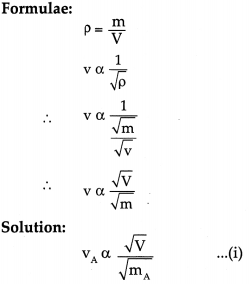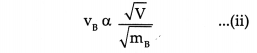Since both bottles are identical hence, the volume is the same, i.e. v
Dividing (j) and (ii),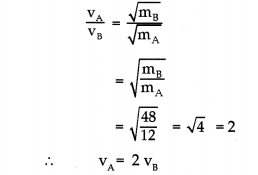(i) Vivacity of sound will be more in bottle A.
(ii) Velocity of sound in bottle A (VA) is twice of that in bottle B (vB)Numerical For Practice

Question 2.
Argon gas is filled in two identical bottles X and Y. The mass of the gas in the two bottles is 5 gm and 25gm respectively. If the speed of sound is the same in both bottles, what conclusions will you draw?
(Temperature of Y is 5 times the temperature of X.)

Type – D

Numerical For Practice

Question 1.
Velocity of sound in air at 0°C is 332nVs. It increases by 0.6ni/s for each °Celsius rise in temperature. At what temperature of ait the velocity will be 359m1s?
45°C

Question 2.
Velocity of sound In air at 0°C is 332m/s It increases by 0.6mIs for each degree Celsius rise In temperature. What will be the velocity of sound at 60°C?
368 rn/s

Define the following:

Question 1.
Wave length (λ)
The distance between two consecutive compressions (or crests) or two consecutive rarefactions (or troughs) is called the wavelength.

Question 2.
Amplitude (A)
The maximum value of pressure or density is called amplitude.

Question 3.
Frequency (υ)
The frequency of a sound wave is defined as the number of complete oscillations of density (or pressure of the medium) per second.Question 4.
Time Period (T)
The time taken for one complete oscillation of pressure or density at a point in the medium is called the time period.

Question 5.
Echo
An echo is the repetition of the original sound because of reflection by some surface.

Question 6.
Transverse waves
Oscillations of the particles of the medium vibrate at right angles to the direction of propagation of the wave are called transverse waves.

Question 7.
longitudinal waves
The particles of the medium oscillate about their central or mean position in a direction parallel to the propagation of wave is called as longitudinal waves.

Question 8.
Velocity of wave
The distance covered by a point on the wave (for example the point of highest density or lowest density) in unit time is the velocity of the sound wave.’

Distinguish between:

Question 1.
Infrasound and Ultrasound

 Infrasound Ultrasound (i) Longitudinal waves whose are below 20 Hz are called Infrasound waves. frequencies Infrasonic or (i) Longitudinal waves whose frequencies lie- above 20,000 Hz are called Ultrasonic or ultrasound waves. (ii) Whales, elephants produce sound in the infrasound range. (ii) Bats produce (30 kHz to 50 kHz) frequency and dolphins produce ultrasound (100 kHz).Question 2.
Transverse waves and Longitudinal waves

 Transverse waves Longitudinal waves (i) Particles of the medium vibrate at right angles to the direction of propagation of the wave. (ii) They produce crests and troughs. (iii) For transverse waves, a wavelength is made up of one crest and one trough. (i) Particles of the medium vibrate parallel to the direction of propagation of the wave. (ii) They produce compression and rarefaction. (iii) For longitudinal waves, a wavelength is made up of one compression and one rarefaction.

Question 3.
Consider two cases
(A) whistle of train (B) roar of a lion

(I) In which case the sound is high pitch?
Whistle of a train is high pitch as compared to roar of a lion, as the frequency is higher.

(II) What is the real cause of sound production? Explain with examples.

• Vibrations in the object are responsible to produce a sound.
• Vibration is a rapid to and fro motion of an object.
• Sometimes the vibrations may be strong enough to be seen by eyes, e.g. string vibrations in string instruments, vibration on mobile phone, blowing air in the cap of your pen by holding it near the lips.

(III) Three sounds 5 Hz, 500 Hz and 50,000 Hz are produced by different sources.
(a) Which sound will be heard by humans?
(b) Which sounds may be produced by bats?
(c) Which sounds may be produced by elephants?
(a) 500 Hz – Humans can hear sounds in the range of 20 Hz-20,000 Hz
(b) 50,000 Hz – Bats produce ultrasonic sounds above 20,000 Hz
(c) 5 Hz – Elephants can produce infrasonic sounds below 20 HzQuestion 6.
Suppose you and your friend are on the moon. Will you be able to hear any sound
Sound waves need a material medium for their propagation. Since there is no atmosphere on the moon, we cannot hear any sound on the moon.

Question 1.
What are the factors on which velocity of sound in gaseous medium depend?
The velocity of sound in a gaseous medium depends on the physical conditions i.e. the temperature, density of the gas and its molecular weight.

1. Temperature (T): The velocity of sound is directly proportional to the square root of the temperature of the medium. This means that increasing the temperature four times doubles the velocity.
$$\text { v } \alpha \sqrt{\mathrm{T}}$$
2. Density(p): The velocity of sound is inversely proportional to the square root of density. Thus, increasing the density four times, reduces the velocity to half its value.
$$\mathrm{v} \alpha \frac{1}{\sqrt{\rho}}$$
3. Molecular weight (M): The velocity sound is inversely proportional to the square root of molecular weight of the gas. Thus, increasing the molecular weight four times, reduces the velocity to haff its value.
$$\mathrm{v} \alpha \frac{1}{\sqrt{\mathrm{M}}}$$

Question 2.
What are the uses of ultrasonic sound?
Uses of ultrasonic sound are as follows:

• For communication between ships at sea.
• To join plastic surfaces together.
• To sterilize liquids like milk by killing the bacteria in it so that the milk keeps for a longer duration.
• Echocardiography which studies heartbeats, is based on ultrasonic waves (Sonography technology).
• To obtain images of internal organs in a human body.
•  In industry to clean intricate parts of machines where hands cannot reach.
• To locate the cracks and faults in metal blocks.Question 3.
Explain with the help of a neat labelled diagram the working of human ear.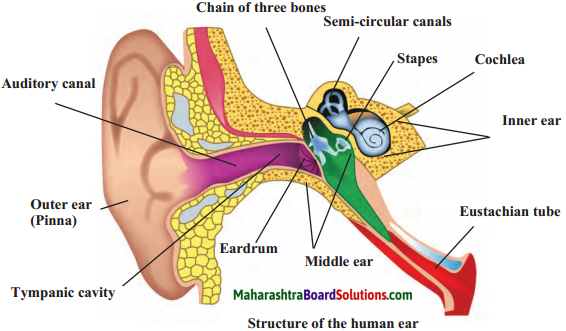• The ear is an important organ of the human body.
• When sound waves fall on the eardrum, it vibrates and these vibrations are converted into electrical signals which travel to the brain through nerves.
• The ear can be divided into three parts:
(a) Outer ear
(b) Middle ear
(c) Inner ear.

(a) Outer ear or Pinna
The outer ear collects the sound waves and passes them through a tube to a cavity in the middle ear. Its peculiar funnel like shape helps to collect and pass sounds into the middle ear.

(b) Middle ear
There’ is a thin membrane in the cavity of the middle ear called the eardrum. When a compression in a sound wave reaches the eardrum, the pressure outside it increases and it gets pushed inwards. The opposite happens when a rarefaction reaches there. The pressure outside decreases and the membrane gets pulled outwards. Thus, sound waves cause vibrations of the membrane.

(c) Inner ear
The auditory nerve connects the inner ear to the brain. The inner ear has a structure resembling the shell of a snail. It is called the cochlea. The cochlea receives the vibrations coming from the membrane and converts them into electrical signals which are sent to the brain through the nerve. The brain analyses these signals.Question 4.
Write a short note on SONAR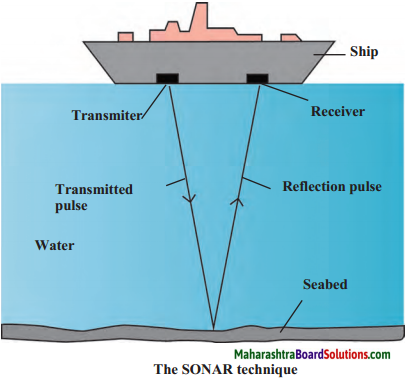(i) SONAR is the short form for Sound Navigation and Ranging. It is used to determine the direction, distance and speed of an underwater object with the help of ultrasonic sound waves. SONAR has a transmitter and a receiver, which are fitted on ships or boats.

(ii) The transmitter produces and transmits ultrasonic sound waves. These waves travel through water, strike underwater objects and get reflected by them. The reflected waves are received by the receiver on the ship.

(iii) The receiver converts the ultrasonic sound into electrical signals and these signals are properly interpreted. The time difference between transmission and reception is noted. This time and the velocity of sound in water give the distance from the ship, of the object which reflects the waves.

(iv) SONAR is used to determine the depth of the sea. SONAR is also used to search underwater hills, valleys, submarines, icebergs, sunken ships etc.

Question 5.
Write a short note on Sonography. How is it misused?
• The gel is used to make proper contact between the skin and the probe so that the full capacity of the ultrasound can be utilized.